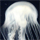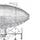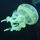General QuestionHow much does the tidal effect of the moon affect a person's weight?

Asked by sundevil (5) June 22nd, 2007

I'm wondering how much it varies with the tidal cycle

Observing members: 0Composing members: 0To not answer your question, far less than water weight changes affect it... =)

bpeoples (2551)“Great Answer” (0) Flag as…Well, in round numbers the moon is 4E8 meters away and has a mass of 7.35E22 kg. G = (6.67E-11 N*m^2/kg^2) so it accelerates you at 6.67E-11 * 7.45E22 / (4E8)^2 = 3.106E-5 N/kg. Your weight due to Earth's gravity (little g) is 9.8 N/kg. So, the moon varies your weight by about plus or minus 0.00032%.

These are rough numbers (particularly, the moon's distance from earth varies quite a bit between apogee and perigee), but that should be correct to well within an order of magnitude.

dfranke (18)“Great Answer” (2) Flag as…or